Fractions and Decimals 2
Set 2 (10 Questions)
Students know and understand that fractions and decimals are two different representations of the same concept to show equivalency, and to refer to parts of a set and parts of a whole. Students can provide examples such as "50 cents is 1/2 dollar, 75 cents is 3/4 of a dollar", etc...
From Mr. Anker Tests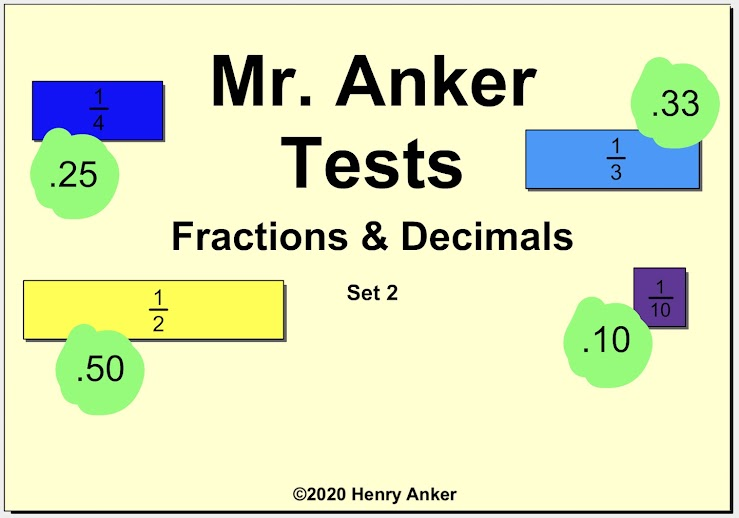Fractions & Decimals Help Slide 1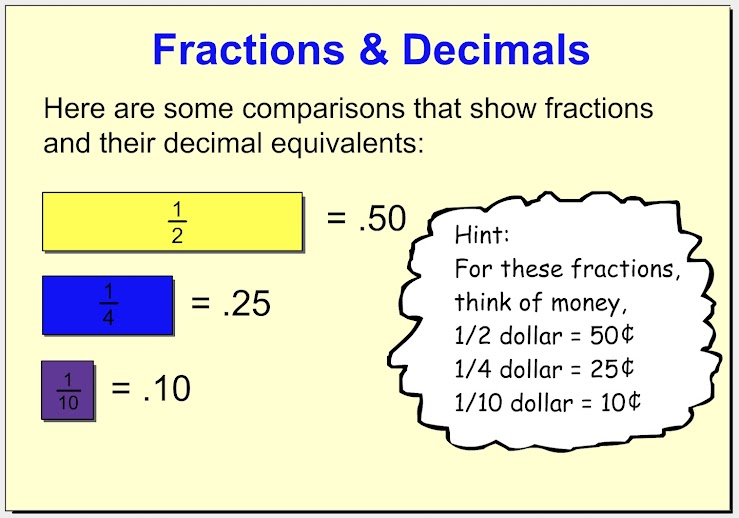Fractions & Decimals Help Slide 2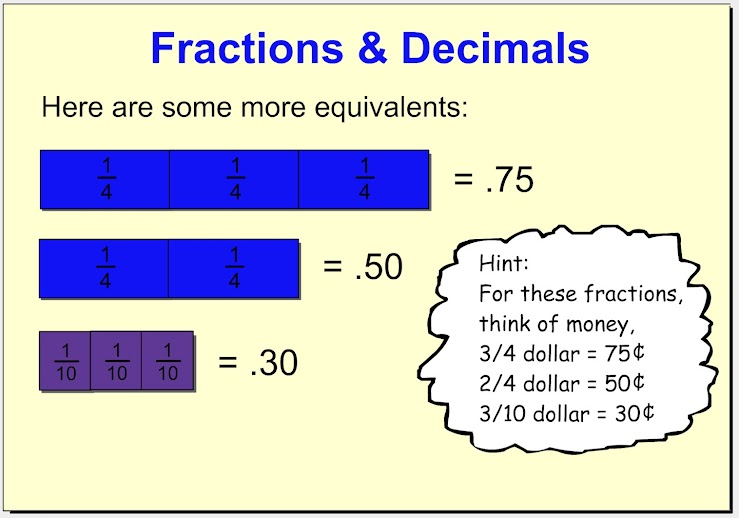1.  Which of decimal values below is equal to 7/10? *
1 point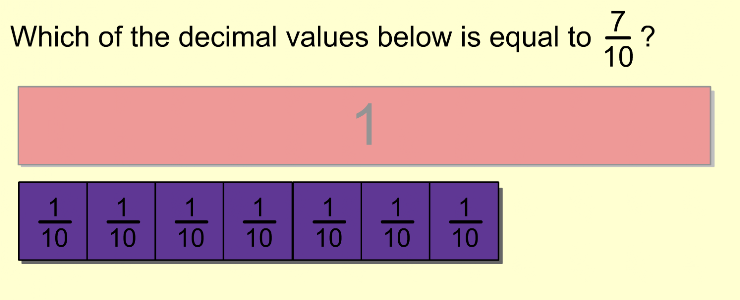2.  Which of decimal values below is equal to 4/10? *
1 point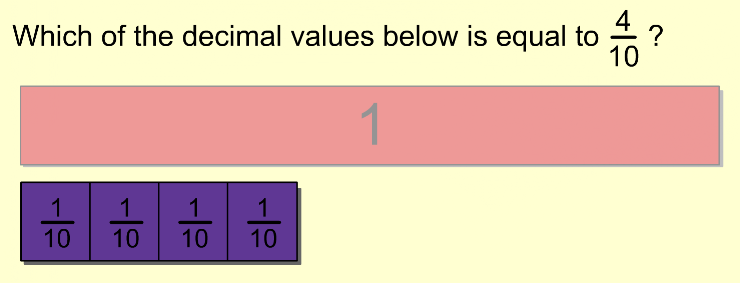3.  Which of decimal values below is equal to 1/3? *
1 point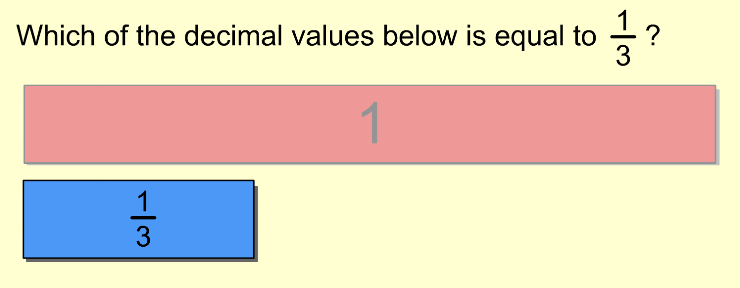4.  Which of decimal values below is equal to 3/3? *
1 point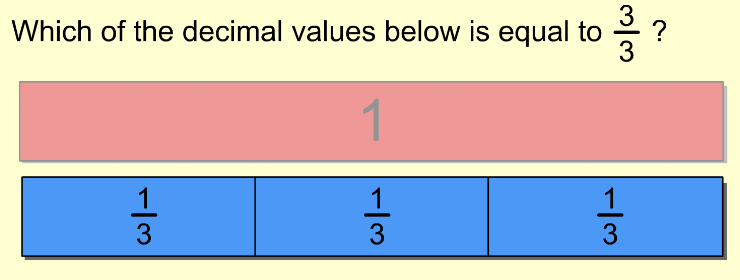5.  Which of decimal values below is equal to 9/10? *
1 point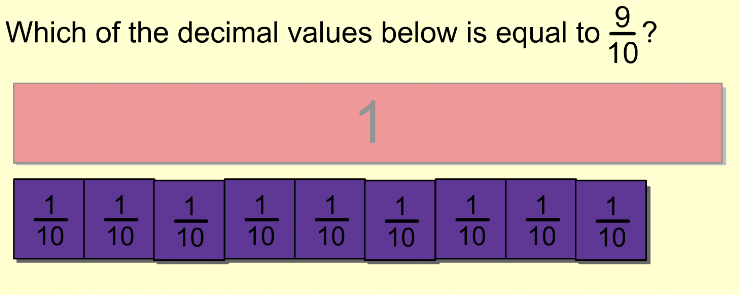6. Which of the decimal values below is equal to 5/10? *
1 point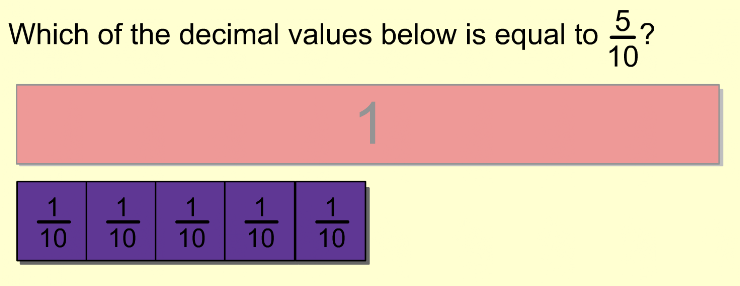7. Which of the decimal values below is equal to 2/4? *
1 point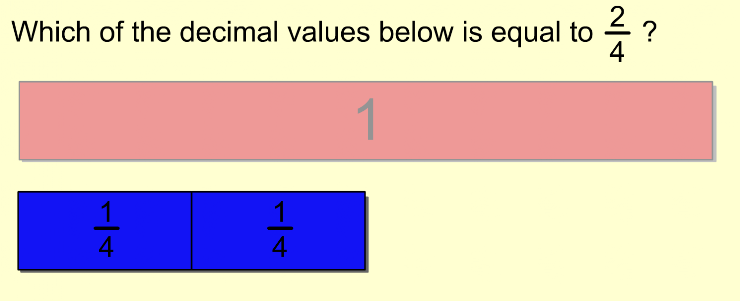8. Which of decimal values below is equal to 16/100? *
1 point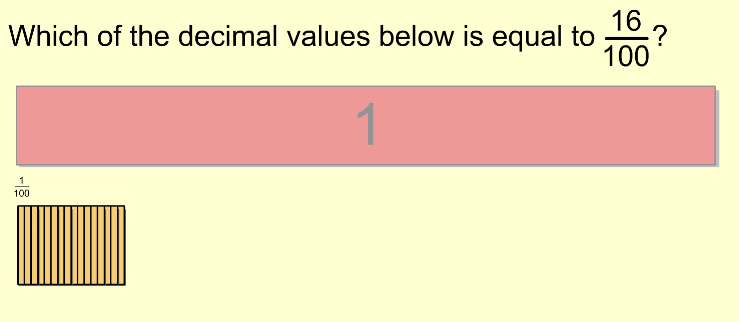9. Which of decimal values below is equal to 25/100? *
1 point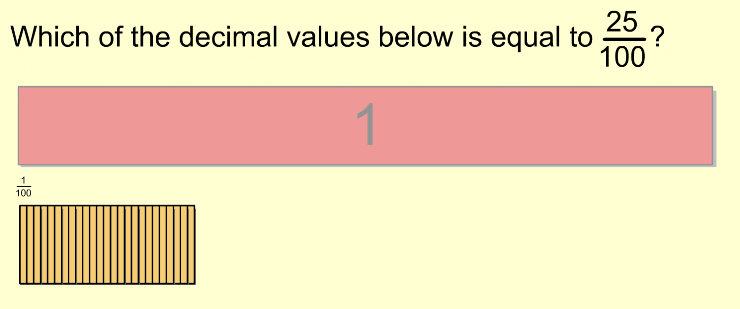10. Which of decimal values below is equal to 105/100? *
1 point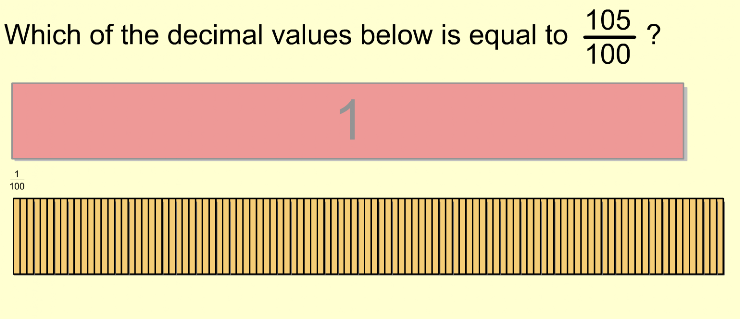Submit
Clear form